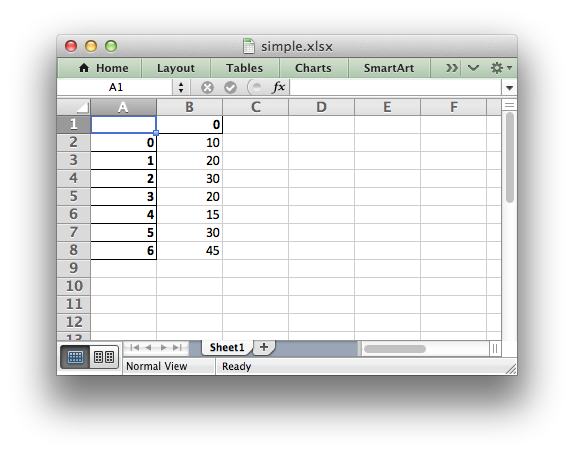# Pandas and XlsxWriter

The following is a simple example of creating a Pandas dataframe and using the `to_excel()` method to write that data out to an Excel file:

```import pandas as pd

# Create a Pandas dataframe from the data.
df = pd.DataFrame([10, 20, 30, 20, 15, 30, 45])

# Create a Pandas Excel writer using XlsxWriter as the engine.
writer = pd.ExcelWriter('simple.xlsx', engine='xlsxwriter')
df.to_excel(writer, sheet_name='Sheet1')

# Close the Pandas Excel writer and output the Excel file.
writer.save()
```

The output from this would look like the following:The option of adding an alternative writer `engine` is only available in Pandas version 0.13 and later.

In order to add a chart to the worksheet we first need to get access to the underlying XlsxWriter Workbook and Worksheet objects.

Continuing on from the above example we do that as follows:

```# Same as above.
writer = pd.ExcelWriter('simple.xlsx', engine='xlsxwriter')
df.to_excel(writer, sheet_name='Sheet1')

# Get the xlsxwriter objects from the dataframe writer object.
workbook  = writer.book
worksheet = writer.sheets['Sheet1']
```

This is equivalent to the following code when using XlsxWriter on its own:

```workbook  = xlsxwriter.Workbook('simple.xlsx')
```

Once we have a Workbook and Worksheet object we can use them to add a chart:

```# Create a chart object.